# Implementing Apriori algorithm in Python

## Implementing Apriori algorithm in Python

Apriori Algorithm is a Machine Learning algorithm which is used to gain insight into the structured relationships between different items involved. The most prominent practical application of the algorithm is to recommend products based on the products already present in the user’s cart. Walmart especially has made great use of the algorithm in suggesting products to it’s users.

Dataset : Groceries data

Implementation of algorithm in Python:
Step 1: Importing the required libraries

 `import` `numpy as np` `import` `pandas as pd` `from` `mlxtend.frequent_patterns ``import` `apriori, association_rules`

 `# Changing the working location to the location of the file` `cd C:\Users\Dev\Desktop\Kaggle\Apriori Algorithm` `# Loading the Data` `data ``=` `pd.read_excel(``'Online_Retail.xlsx'``)` `data.head()`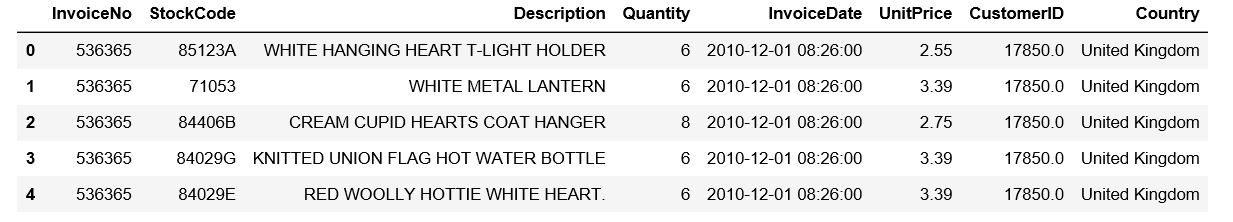`# Exploring the columns of the data` `data.columns`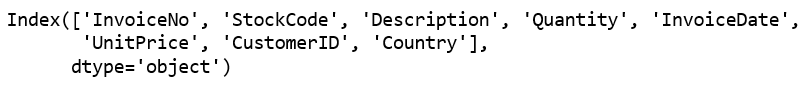`# Exploring the different regions of transactions` `data.Country.unique()`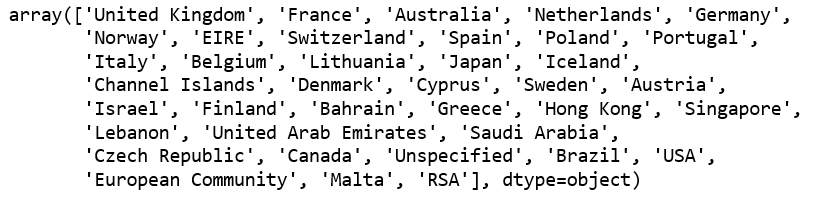Step 3: Cleaning the Data

 `# Stripping extra spaces in the description` `data[``'Description'``] ``=` `data[``'Description'``].``str``.strip()` `# Dropping the rows without any invoice number` `data.dropna(axis ``=` `0``, subset ``=``[``'InvoiceNo'``], inplace ``=` `True``)` `data[``'InvoiceNo'``] ``=` `data[``'InvoiceNo'``].astype(``'str'``)` `# Dropping all transactions which were done on credit` `data ``=` `data[~data[``'InvoiceNo'``].``str``.contains(``'C'``)]`

Step 4: Splitting the data according to the region of transaction

 `# Transactions done in France` `basket_France ``=` `(data[data[``'Country'``] ``=``=``"France"``]` `          ``.groupby([``'InvoiceNo'``, ``'Description'``])[``'Quantity'``]` `          ``.``sum``().unstack().reset_index().fillna(``0``)` `          ``.set_index(``'InvoiceNo'``))` `# Transactions done in the United Kingdom` `basket_UK ``=` `(data[data[``'Country'``] ``=``=``"United Kingdom"``]` `          ``.groupby([``'InvoiceNo'``, ``'Description'``])[``'Quantity'``]` `          ``.``sum``().unstack().reset_index().fillna(``0``)` `          ``.set_index(``'InvoiceNo'``))` `# Transactions done in Portugal` `basket_Por ``=` `(data[data[``'Country'``] ``=``=``"Portugal"``]` `          ``.groupby([``'InvoiceNo'``, ``'Description'``])[``'Quantity'``]` `          ``.``sum``().unstack().reset_index().fillna(``0``)` `          ``.set_index(``'InvoiceNo'``))` `basket_Sweden ``=` `(data[data[``'Country'``] ``=``=``"Sweden"``]` `          ``.groupby([``'InvoiceNo'``, ``'Description'``])[``'Quantity'``]` `          ``.``sum``().unstack().reset_index().fillna(``0``)` `          ``.set_index(``'InvoiceNo'``))`

Step 5: Hot encoding the Data

 `# Defining the hot encoding function to make the data suitable` `# for the concerned libraries` `def` `hot_encode(x):` `    ``if``(x<``=` `0``):` `        ``return` `0` `    ``if``(x>``=` `1``):` `        ``return` `1` `# Encoding the datasets` `basket_encoded ``=` `basket_France.applymap(hot_encode)` `basket_France ``=` `basket_encoded` `basket_encoded ``=` `basket_UK.applymap(hot_encode)` `basket_UK ``=` `basket_encoded` `basket_encoded ``=` `basket_Por.applymap(hot_encode)` `basket_Por ``=` `basket_encoded` `basket_encoded ``=` `basket_Sweden.applymap(hot_encode)` `basket_Sweden ``=` `basket_encoded`

Step 6: Building the models and analyzing the results
a) France:

 `# Building the model` `frq_items ``=` `apriori(basket_France, min_support ``=` `0.05``, use_colnames ``=` `True``)` `# Collecting the inferred rules in a dataframe` `rules ``=` `association_rules(frq_items, metric ``=``"lift"``, min_threshold ``=` `1``)` `rules ``=` `rules.sort_values([``'confidence'``, ``'lift'``], ascending ``=``[``False``, ``False``])` `print``(rules.head())`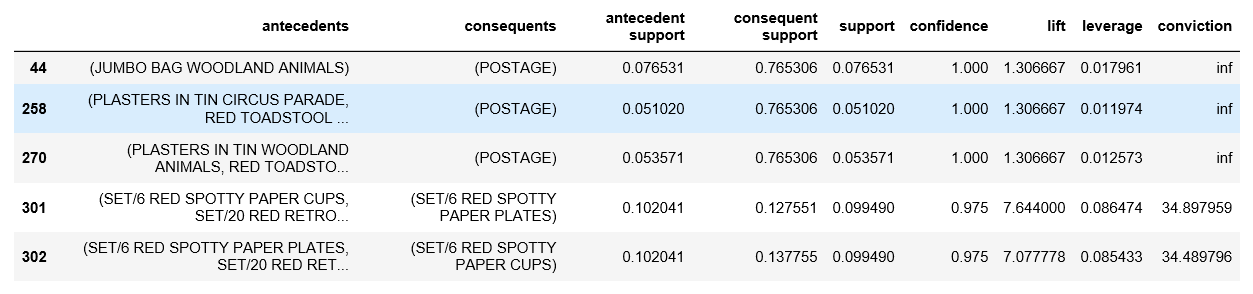From the above output, it can be seen that paper cups and paper and plates are bought together in France. This is because the French have a culture of having a get-together with their friends and family atleast once a week. Also, since the French government has banned the use of plastic in the country, the people have to purchase the paper-based alternatives.
b) United Kingdom:

 `frq_items ``=` `apriori(basket_UK, min_support ``=` `0.01``, use_colnames ``=` `True``)` `rules ``=` `association_rules(frq_items, metric ``=``"lift"``, min_threshold ``=` `1``)` `rules ``=` `rules.sort_values([``'confidence'``, ``'lift'``], ascending ``=``[``False``, ``False``])` `print``(rules.head())`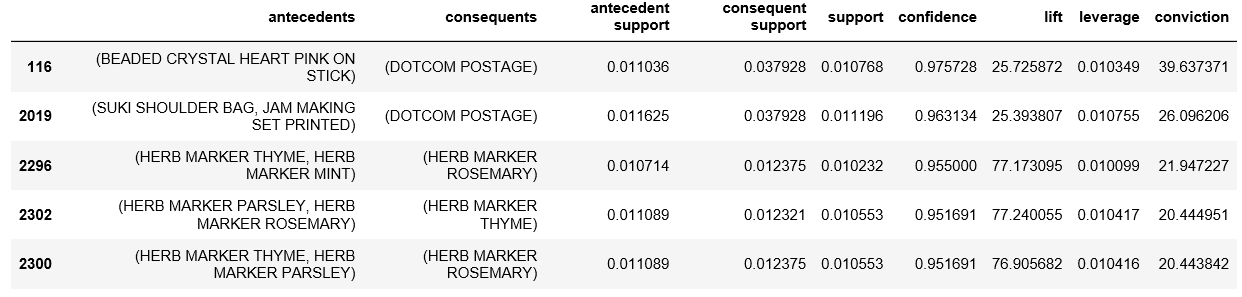If the rules for British transactions are analyzed a little deeper, it is seen that the British people buy different colored tea-plates together. A reason behind this may be because typically the British enjoy tea very much and often collect different colored tea-plates for different occasions.
c) Portugal:

 `frq_items ``=` `apriori(basket_Por, min_support ``=` `0.05``, use_colnames ``=` `True``)` `rules ``=` `association_rules(frq_items, metric ``=``"lift"``, min_threshold ``=` `1``)` `rules ``=` `rules.sort_values([``'confidence'``, ``'lift'``], ascending ``=``[``False``, ``False``])` `print``(rules.head())`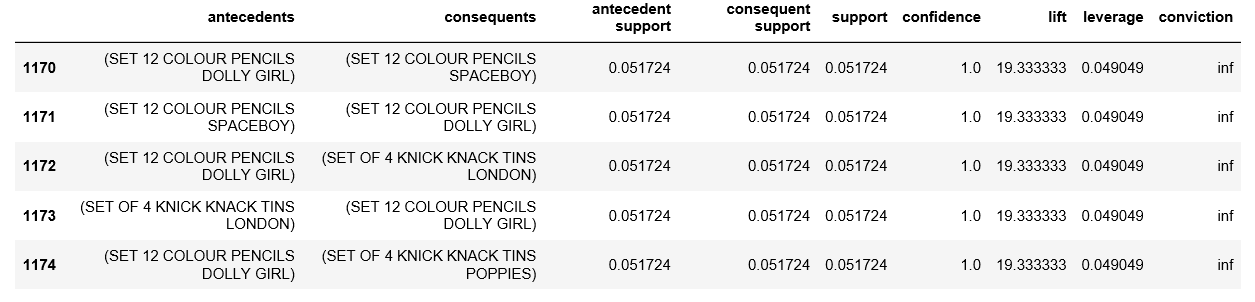On analyzing the association rules for Portuguese transactions, it is observed that Tiffin sets (Knick Knack Tins) and color pencils. These two products typically belong to a primary school going kid. These two products are required by children in school to carry their lunch and for creative work respectively and hence are logically make sense to be paired together.
d) Sweden:

 `frq_items ``=` `apriori(basket_Sweden, min_support ``=` `0.05``, use_colnames ``=` `True``)` `rules ``=` `association_rules(frq_items, metric ``=``"lift"``, min_threshold ``=` `1``)` `rules ``=` `rules.sort_values([``'confidence'``, ``'lift'``], ascending ``=``[``False``, ``False``])` `print``(rules.head())`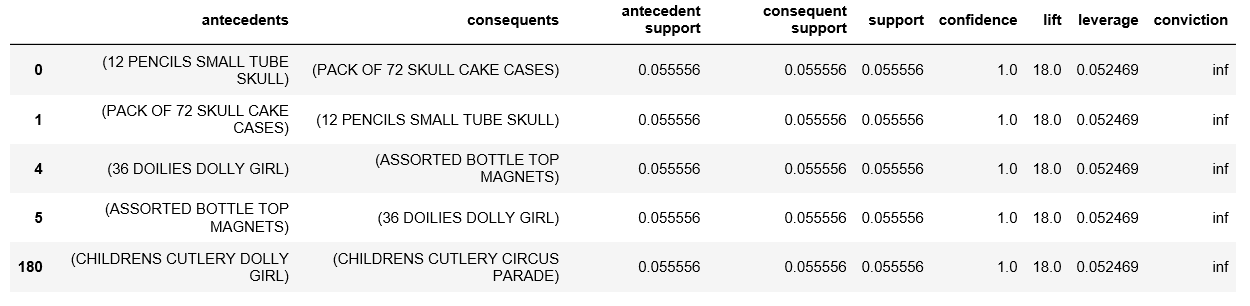On analyzing the above rules, it is found that boys’ and girls’ cutlery are paired together. This makes practical sense because when a parent goes shopping for cutlery for his/her children, he/she would want the product to be a little customized according to the kid’s wishes.

Last Updated on November 13, 2021 by admin

## Create a new column in Pandas DataFrame based on the existing columnsCreate a new column in Pandas DataFrame based on the existing columns

Create a new column in Pandas DataFrame based on the existing columns While working with

## Change data type of given numpy arrayChange data type of given numpy array

Change data type of given numpy array In this post, we are going to see

## Python program to find second largest number in a listPython program to find second largest number in a list

Given a list of numbers, the task is to write a Python program to find

## Exporting a Pandas DataFrame to an Excel fileExporting a Pandas DataFrame to an Excel file

Exporting a Pandas DataFrame to an Excel file Let us see how to export a

## How to read from a file in PythonHow to read from a file in Python

How to read from a file in Python Python provides inbuilt functions for creating, writing

## How to convert Dictionary to Pandas Dataframe?How to convert Dictionary to Pandas Dataframe?

Pandas is a popular library for data manipulation and analysis in Python. It provides various

## Python GUI – tkinterPython GUI – tkinter

Python GUI – tkinter Python offers multiple options for developing GUI (Graphical User Interface). Out

## randint() Function in Pythonrandint() Function in Python

randint() Function in Python randint() is an inbuilt function of the random module in Python3. The random module